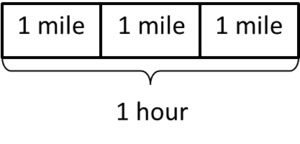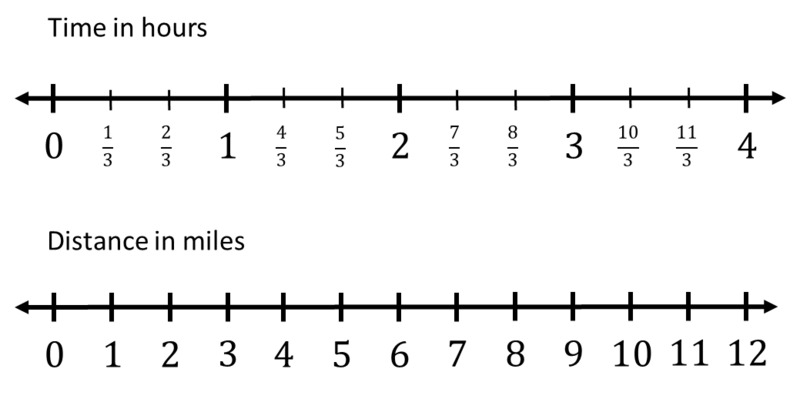# Walk-a-thon 1

Alignments to Content Standards: 6.RP.A.3.a 6.RP.A.3.b

Julianna participated in a walk-a-thon to raise money for cancer research. She recorded the total distance she walked at several different points in time, but a few of the entries got smudged and can no longer be read. The times and distances that can still be read are listed in the table below.

1. Assume Julianna walked at a constant speed. Complete the table and plot Julianna’s progress in the coordinate plane.
2. How fast was Julianna walking in miles per hour? How long did it take Julianna to walk one mile?
3. Next year Julianna is planning to walk for seven hours. If she walks at the same speed next year, how many miles will she walk?
Time in hrs Miles walked
1
2 6
12
5

## IM Commentary

In this task, students are given information about a context where there is a proportional relationship between two quantities in a table that has missing values. Students need to fill in the missing values, plot the corresponding points in the coordinate plane, and find the two unit rates that are associated with this proportional relationship. They then use these mathematical tools to make predictions about the future.

In 7th grade, students will deepen their understanding of the connections between tables, graphs, and verbal descriptions that represent proportional relationships and identify the unit rate $r$ as the vertical coordinate of a point on the graph with horizontal coordinate 1. For a version of this task that shows the 7th grade expectations, please see:

7.RP Walk-a-thon 2 http://www.illustrativemathematics.org/illustrations/1526

The Standards for Mathematical Practice focus on the nature of the learning experiences by attending to the thinking processes and habits of mind that students need to develop in order to attain a deep and flexible understanding of mathematics. Certain tasks lend themselves to the demonstration of specific practices by students. The practices that are observable during exploration of a task depend on how instruction unfolds in the classroom. While it is possible that tasks may be connected to several practices, the commentary will spotlight one practice connection in depth. Possible secondary practice connections may be discussed but not in the same degree of detail.

Mathematical Practice 5, "Use appropriate tools strategically," emphasizes a student’s ability to consider a tool’s usefulness and constraints as well as knowing how to use it appropriately. During this task, students engage in MP.5 by considering the connections among tables, graphs, and verbal descriptions (tools) to find the unit rate associated with the proportional relationship. They extend their understanding by using these same tools to predict how many miles Julianna will walk next year if she maintains the same speed. Although this particular task directs students to use specific tools, it does not prevent them from trying additional tools. A discussion regarding what other tools may be efficient and appropriate to use and why you might use a particular tool would be beneficial.

## Solution

1. We see that the only complete time-distance pair indicates that Julianna walked 6 miles in 2 hours. If she walked at a constant speed, we can conclude that Julianna walked 3 miles in 1 hour. Using this speed, we can find the remaining values in the table by multiplying the hours she walked by 3 (or, alternatively, by entering 3 in row next to 1 and then adding 3 for every hour walked). We get the following table.

Time in hrs Miles walked
1 3
2 6
3 9
4 12
5 15

Let's plot her progress in the coordinate plane by having her time, in hours, be represented on the horizontal axis, and the number of miles she walked be represented on the vertical axis. Using the rows in the table above as our coordinate points, we get the following graph.2. We found in part (a) that Julianna walks 3 miles in 1 hour.Looking at the picture above, we can see that if she walks 3 miles in one hour, and she is walking at a constant speed, then it will take her $\frac13$ of an hour to walk 1 mile.

We can also look at a double number line.We can see on the double number line that 1 hour corresponds to three miles and $\frac13$ hour corresponds to 1 mile.

So, we find that it took Julianna $\frac 13$ hours, or 20 minutes, to walk 1 mile.

3. We found in parts (a) and (b) that Julianna’s rate of travel was 3 miles per hour. If this stayed constant, we can find how many miles she would walk in 7 hours by extending our table.

Time in hrs Miles walked
1 3
2 6
3 9
4 12
5 15
6 18
7 21

Thus, we see that in 7 hours, Julianna will walk 21 miles at that rate.